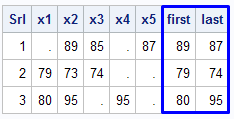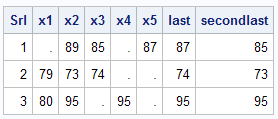# SAS : Second Non-Missing Value

This tutorial demonstrates how to get second or second last non-missing value across rows. In SAS, there is a function called COALESCE to extract first non-missing value in a row. So it is an easy job to fetch first or last non-missing value but tricky to pull second or second last non-missing value. It is mostly used to see the customer behavior over past 2-3 periods before the account gets closed.

Example 1 : Extract First or Last Non-Missing ValueExample : COALESCE Function
Dummy Dataset
data example;
input Srl x1-x5;
cards;
1 . 89 85 . 87
2 79 73 74 . .
3 80 95 . 95 .
;
run;
data out;
set example;
first = coalesce(of x1-x5);
last = coalesce(of x5-x1);
proc print noobs;
run;

The coalesce(of x1-x5) would check first non-missing in variables starting from x1 to x5 across rows and returns the values in each rows. To get last value in a row, just reverse the order of variables. The coalesce(of x5-x1) would return first non-missing in variables x5, x4, x3, x2, x1. So, it would be indirectly last value as order of variables is opposite.

Example 2 : Extract Second Non-Missing Value

data out;
set example;
array value x1-x5;
array nvalue(5) _temporary_;
first = coalesce(of value(*));
index = whichn(first, of value(*));
do i = 1 to dim(value);
if i = index then nvalue(i) = .;
else nvalue(i)= value(i);
end;
drop i index;
second = coalesce(of nvalue(*));
proc print noobs;
run;Get Second Non-Missing Value
How it works -
1. First, create an array for variables from which we want to extract second non-missing value
2. The idea is to make first non-missing value missing so that we can pull second non-missing value
3. First, we need to create a temporary array of 5 variables in which we can store value.
4. The function WHICHN is used to find the column position of first non-missing value. For example, it returns 2 for row 1 values.
5. The DO LOOP is used to run the above steps iteratively in loop.

Example 3 : Pull Second Last Non-Missing Value

data out2;
set example;
array value x5-x1;
array nvalue(5) _temporary_;
last = coalesce(of value(*));
index = whichn(last, of value(*));
do i = 1 to dim(value);
if i = index then nvalue(i) = .;
else nvalue(i)= value(i);
end;
drop i index;
secondlast = coalesce(of nvalue(*));
proc print noobs;
run;SAS : Second Last Non-Missing
The only difference between second and third example is how variables are defined in array 'value'. In the example 3, we have reversed the position of variables to fetch second last non-missing value.

Related Posts1.